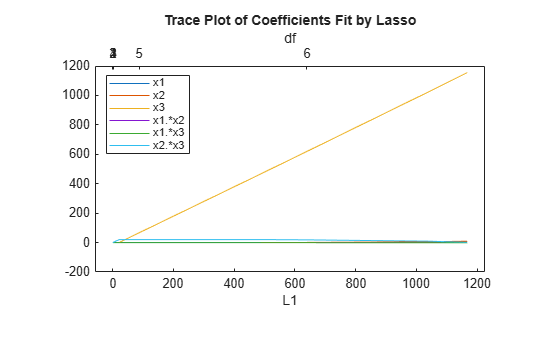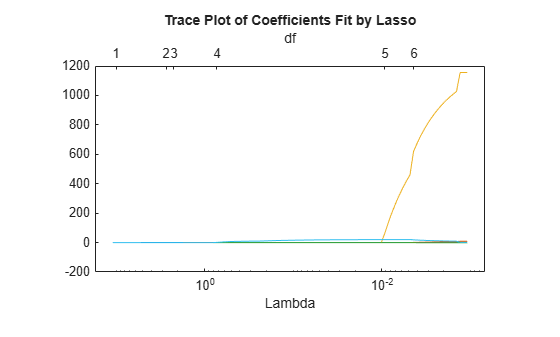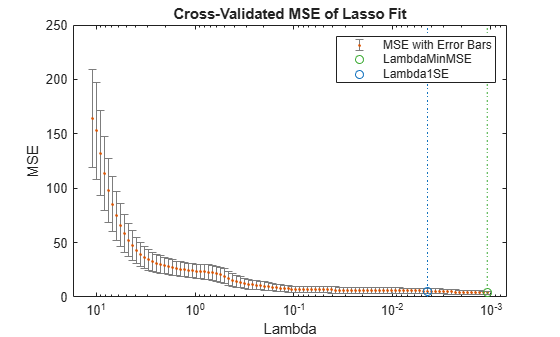# lassoPlot

Trace plot of lasso fit

## Syntax

``lassoPlot(B)``
``lassoPlot(B,FitInfo)``
``lassoPlot(B,FitInfo,Name=Value)``
``````[ax,figh] = lassoPlot(___)``````

## Description

````lassoPlot(B)` creates a trace plot of the values in `B` against the L1 norm of `B`.```
````lassoPlot(B,FitInfo)` creates a plot with type depending on the data type of `FitInfo` and the value, if any, of the `PlotType` name-value argument.```

example

````lassoPlot(B,FitInfo,Name=Value)` creates a plot with additional options specified by one or more name-value arguments.```
``````[ax,figh] = lassoPlot(___)```, for any previous input syntax, returns the axes `ax` and the figure window `figh` that contain the plot of the lasso fit.```

## Examples

collapse all

`load acetylene`

Prepare the design matrix for lasso fit with interactions. The `x2fx` function returns the quadratic model in the order of a constant term, linear terms and interaction terms: constant term, `x1`, `x2`, `x3`, `x1.*x2`, `x1.*x3`, and `x2.*x3.`

```X = [x1 x2 x3]; D = x2fx(X,"interaction"); D(:,1) = []; % No constant term```

Fit a regularized model of the data using the `lasso` function.

`B = lasso(D,y);`

Plot the lasso fits with labeled coefficients by using the `PredictorNames` name-value argument. Each line represents a trace of the values in B for a single predictor variable: `x1`, `x2`, `x3`, `x1.*x2`, `x1.*x3`, and `x2.*x3`.

```lassoPlot(B,PredictorNames=["x1" "x2" "x3" "x1.*x2" "x1.*x3" "x2.*x3"]); legend("show",Location="NorthWest")````load acetylene`

Prepare the data for lasso fit with interactions.

```X = [x1 x2 x3]; D = x2fx(X,"interaction"); D(:,1) = []; % No constant term```

Fit a regularized model of the data using the `lasso` function.

`[B,FitInfo] = lasso(D,y);`

Plot the fits with the `Lambda` plot type and logarithmic scaling.

`lassoPlot(B,FitInfo,PlotType="Lambda",XScale="log");`Visually examine the cross-validated error of various levels of regularization.

`load acetylene`

Create a design matrix with interactions and no constant term.

```X = [x1 x2 x3]; D = x2fx(X,"interaction"); D(:,1) = []; % No constant term```

Construct the lasso fit using 10-fold cross-validation. Include the `FitInfo` output so you can plot the result.

```rng default % For reproducibility [B,FitInfo] = lasso(D,y,CV=10);```

Plot the cross-validated fits. The green circle and dotted line locate the `Lambda` with minimum cross-validation error. The blue circle and dotted line locate the point with minimum cross-validation error plus one standard error.

```lassoPlot(B,FitInfo,PlotType="CV"); legend("show")```## Input Arguments

collapse all

Coefficients of a sequence of regression fits, specified as a numeric matrix of size p-by-L, where p is the number of predictors and L is the number of regularization coefficients `Lambda`. You can calculate `B` using the `lasso` or `lassoglm` function.

Fit information of the generalized linear models, specified as a structure or a numeric vector.

• When `FitInfo` is a structure, such as returned from the `lasso` or `lassoglm` function, the `lassoPlot` function creates a plot based on the `PlotType` name-value argument.

• When `FitInfo` is a vector, the `lassoPlot` function forms the x-axis of the plot from the values in `FitInfo`. The length of `FitInfo` must equal the number of columns of `B`.

### Name-Value Arguments

Specify optional pairs of arguments as `Name1=Value1,...,NameN=ValueN`, where `Name` is the argument name and `Value` is the corresponding value. Name-value arguments must appear after other arguments, but the order of the pairs does not matter.

Example: `lassoPlot(B,XScale="log")` uses a logarithmic scaled x-axis.

Before R2021a, use commas to separate each name and value, and enclose `Name` in quotes.

Example: `lassoPlot(B,"XScale","log")` uses a logarithmic scaled x-axis.

Parent axes in which to draw the plot, specified as an `Axes` object. If you do not specify `Parent`, then `lassoPlot` creates the plot using the current axes. For more information on creating an `Axes` object, see `axes`.

Plot type, specified as `"L1"`, `"Lambda"`, or `"CV"`. The `PlotType` argument applies when you specify the `FitInfo` argument.

`PlotType`Plot
`"L1"``lassoPlot` creates the x-axis from the L1 norm of the coefficients in `B`. The x-axis at the top of the plot contains the degrees of freedom (`df`), meaning the number of nonzero coefficients of `B`.

`"Lambda"`

`lassoPlot` creates the x-axis from the `Lambda` field of `FitInfo`. The x-axis at the top of the plot contains the degrees of freedom (`df`), meaning the number of nonzero coefficients of `B`.

When you choose this value, `FitInfo` must be a structure.

`"CV"`

• For each `Lambda`, `lassoPlot` plots an estimate of the mean squared prediction error on new data for the model fitted by `lasso` with that value of `Lambda`.

• `lassoPlot` plots error bars for the estimates.

When you choose this value, `FitInfo` must be a cross-validated structure.

If you include a cross-validated `FitInfo` structure, `lassoPlot` also indicates two specific `Lambda` values with green and blue dashed lines.

• A green, dashed line indicates the value of `Lambda` with a minimum cross-validated mean squared error (MSE).

• A blue, dashed line indicates the greatest `Lambda` that is within one standard error of the minimum MSE. This Lambda value makes the sparsest model with relatively low MSE.

To display the label for each plot in the legend of the figure, type `legend("show")` in the Command Window.

Predictor names, specified as a string array or cell array of character vectors. The predictor names label each coefficient of `B`. If the length of the `PredictorNames` argument is less than the number of rows of `B`, the remaining labels are padded with default values.

If you do not specify this argument, but you specify the `FitInfo` argument as a structure and the `PredictorNames` field of the structure is not empty (`{}`), then the `lassoPlot` function uses those predictor names instead of default values.

To display the legend with the predictor name as the label for each plot, type `legend("show")` in the Command Window.

Scale of values along the x-axis, specified as one of these values.

• `"linear"` — Linear x-axis. This is the default value when the `PlotType` argument is `"L1"` or `"Lambda"`.

• `"log"` — Logarithmic scaled x-axis. This is the default value when the `PlotType` argument is `"CV"`.

Data Types: `string` | `char`

## Output Arguments

collapse all

Axes of the plot, returned as an `Axes` object. For more information, see Axes Appearance.

Figure window of the plot, returned as a `Figure` object. For more information, see Special Object Identifiers.

## Version History

Introduced in R2011b